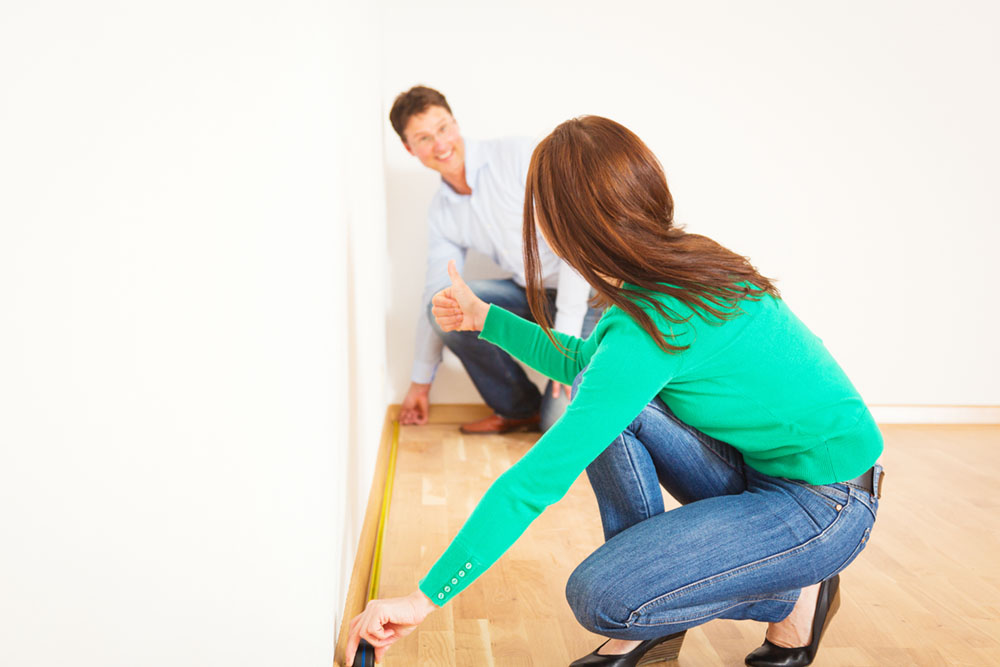# Calculating the Square Footage of a House

All Metro Team May 11, 2023Calculating the square footage of a house is an important step in determining the value of a property, as well as understanding the amount of living space that is available. Square footage is also used to determine how much material you’ll need for renovations or upgrades. In addition, it’s a key factor that many homebuyers consider when making a purchase. Here are the steps you need to follow to calculate the square footage of a house.

### Make a rough sketch of the house

Before you begin measuring the rooms, it is a good idea to make a rough sketch of the house on a piece of paper. This will help you keep track of which rooms you have measured and which ones you still need to measure.

### Measure each room

To begin, measure the length and width of each room. Measure from the baseboard or wall to the opposite wall. Be sure to measure at the widest points of the room. Jot down those measurements on your sketch.

### Calculate the square footage of each room

To calculate the square footage of each room, simply multiply the length by the width. For example, if the room is 12 feet long and 10 feet wide, then the total square footage would be 120 (10 x 12 = 120). Write down the square footage of each room on your sketch.

### Add up the total square footage

Once you have calculated the square footage of each room, add up all the square footage numbers to get the total square footage of the house. For example, if there are three rooms with a square footage of 120, 150, and 180 square feet, the total square footage would be 450 square feet (120 + 150 + 180 = 450).

### Account for any additional living spaces

In addition to measuring the rooms, it is also important to account for any additional living spaces that may not be considered separate rooms. This includes hallways, staircases, and closets. To measure these spaces, simply measure the length and width as you would for a room and then calculate the square footage. Add this to your total square footage.

### Round up to the nearest square foot

Once you have calculated the total square footage of the house, it is a good idea to round up to the nearest square foot. This will give you a more accurate estimate of the amount of living space in the house.

### Know what not to include

Lastly, it is important to note that some areas of the house should not be included when calculating square footage. For example, any areas that are not enclosed or heated, such as a garage, porch, or unfinished basement, should not be included in the square footage calculation. Additionally, any areas with a ceiling height of fewer than seven feet should not be included.

Calculating the square footage of a house can be a time-consuming process, but it is an important step in understanding the value and livable space of a property. By following the steps outlined above, you can accurately calculate the square footage of a house. You can then use this information to make informed decisions about buying, selling, or renovating a property.

Compliments of Virtual Results